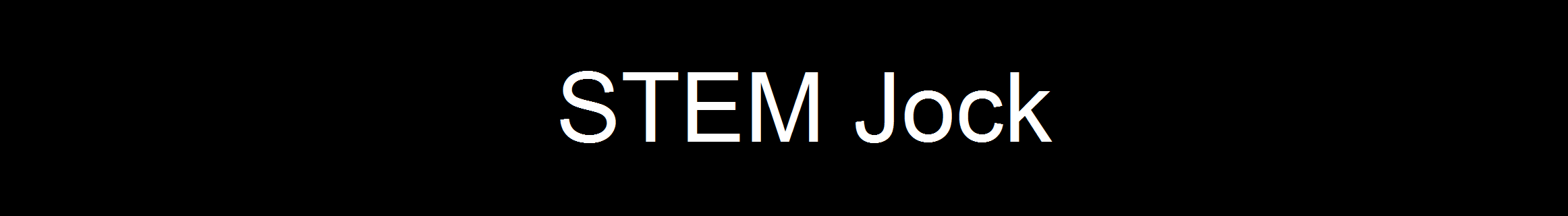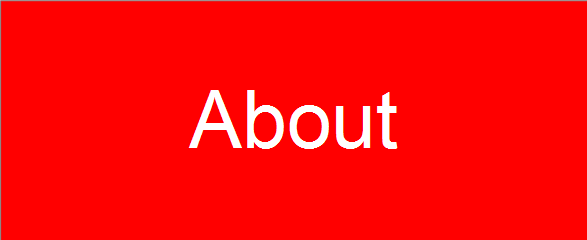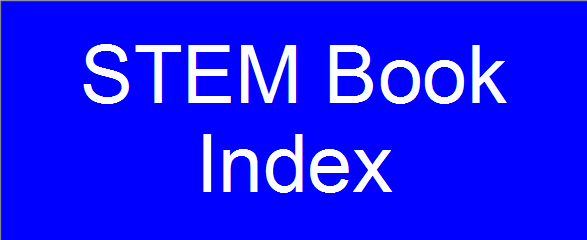• Chapter P: Preparation for Calculus
• Section P.1: Graphs and Models
• Section P.2: Linear Models and Rates of Change
• Section P.3: Functions and Their Graphs
• Section P.4: Review of Trigonometric Functions

• Chapter 1: Limits and Their Properties
• Section 1.1: A Preview of Calculus
• Section 1.2: Finding Limits Graphically and Numerically
• Section 1.3: Evaluating Limits Analytically
• Section 1.4: Continuity and One-Sided Limits
• Section 1.5: Infinite Limits

• Chapter 2: Differentiation
• Section 2.1: The Derivative and the Tangent Line Problem
• Section 2.2: Basic Differentiation Rules and Rates of Change
• Section 2.3: Product and Quotient Rules and Higher-Order Derivatives
• Section 2.4: The Chain Rule
• Section 2.5: Implicit Differentiation
• Section 2.6: Related Rates

• Chapter 3: Applications of Differentiation
• Section 3.1: Extrema on an Interval
• Section 3.2: Rolle's Theorem and the Mean Value Theorem
• Section 3.3: Increasing and Decreasing Functions and the First Derivative Test
• Section 3.4: Concavity and the Second Derivative Test
• Section 3.5: Limits at Infinity
• Section 3.6: A Summary of Curve Sketching
• Section 3.7: Optimization Problems
• Section 3.8: Newton's Method
• Section 3.9: Differentials

• Chapter 4: Integration
• Section 4.1: Antiderivatives and Indefinite Integration
• Section 4.2: Area
• Section 4.3: Riemann Sums and Definite Integrals
• Section 4.4: The Fundamental Theorem of Calculus
• Section 4.5: Integration by Substitution

• Chapter 5: Logarithmic, Exponential, and Other Transcendental Functions
• Section 5.1: The Natural Logarithmic Function: Differentiation
• Section 5.2: The Natural Logarithmic Function: Integration
• Section 5.3: Inverse Functions
• Section 5.4: Exponential Functions: Differentiation and Integration
• Section 5.5: Bases Other than e and Applications
• Section 5.6: Indeterminate Forms and L'Hopital's Rule
• Section 5.7: Inverse Trigonometric Functions: Differentiation
• Section 5.8: Inverse Trigonometric Functions: Integration
• Section 5.9: Hyperbolic Functions
• Chapter 6: Differential Equations
• Section 6.1: Slope Fields and Euler's Method
• Section 6.2: Growth and Decay
• Section 6.3: Separation of Variables and the Logistic Equation
• Section 6.4: First-Order Linear Differential Equations

• Chapter 7: Applications of Integration
• Section 7.1: Area of a Region Between Two Curves
• Section 7.2: Volume: The Disk Method
• Section 7.3: Volume: The Shell Method
• Section 7.4: Arc Length and Surfaces of Revolution
• Section 7.5: Work
• Section 7.6: Moments, Centers of Mass, and Centroids
• Section 7.7: Fluid Pressure and Fluid Force

• Chapter 8: Integration Techniques and Improper Integrals
• Section 8.1: Basic Integration Rules
• Section 8.2: Integration by Parts
• Section 8.3: Trigonometric Integrals
• Section 8.4: Trigonometric Substitution
• Section 8.5: Partial Fractions
• Section 8.6: Numerical Integration
• Section 8.7: Integration by Tables and Other Integration Techniques
• Section 8.8: Improper Integrals

• Chapter 9: Infinite Series
• Section 9.1: Sequences
• Section 9.2: Series and Convergence
• Section 9.3: The Integral Test and p-Series
• Section 9.4: Comparisons of Series
• Section 9.5: Alternating Series
• Section 9.6: The Ratio and Root Tests
• Section 9.7: Taylor Polynomials and Approximations
• Section 9.8: Power Series
• Section 9.9: Representation of Functions by Power Series
• Section 9.10: Taylor and Maclaurin Series

• Chapter 10: Conics, Parametric Equations, and Polar Coordinates
• Section 10.1: Conics and Calculus
• Section 10.2: Plane Curves and Parametric Equations
• Section 10.3: Parametric Equations and Calculus
• Section 10.4: Polar Coordinates and Polar Graphs
• Section 10.5: Area and Arc Length in Polar Coordinates
• Section 10.6: Polar Equations of Conics and Kepler's Laws

• Chapter 11: Vectors and the Geometry of Space
• Section 11.1: Vectors in the Plane
• Section 11.2: Space Coordinates and Vectors in Space
• Section 11.3: The Dot Product of Two Vectors
• Section 11.4: The Cross Product of Two Vectors in Space
• Section 11.5: Lines and Planes in Space
• Section 11.6: Surfaces in Space
• Section 11.7: Cylindrical and Spherical Coordinates
• Chapter 12: Vector-Valued Functions
• Section 12.1: Vector-Valued Functions
• Section 12.2: Differentiation and Integration of Vector-Valued Functions
• Section 12.3: Velocity and Acceleration
• Section 12.4: Tangent Vectors and Normal Vectors
• Section 12.5: Arc Length and Curvature

• Chapter 13: Functions of Several Variables
• Section 13.1: Introduction to Functions of Several Variables
• Section 13.2: Limits and Continuity
• Section 13.3: Partial Derivatives
• Section 13.4: Differentials
• Section 13.5: Chain Rules for Functions of Several Variables
• Section 13.6: Directional Derivatives and Gradients
• Section 13.7: Tangent Planes and Normal Lines
• Section 13.8: Extrema of Functions of Two Variables
• Section 13.9: Applications of Extrema
• Section 13.10: Lagrange Multipliers

• Chapter 14: Multiple Integration
• Section 14.1: Iterated Integrals and Area in the Plane
• Section 14.2: Double Integrals and Volume
• Section 14.3: Change of Variables: Polar Coordinates
• Section 14.4: Center of Mass and Moments of Inertia
• Section 14.5: Surface Area
• Section 14.6: Triple Integrals and Applications
• Section 14.7: Triple Integrals in Other Coordinates
• Section 14.8: Change of Variables: Jacobians

• Chapter 15: Vector Analysis
• Section 15.1: Vector Fields
• Section 15.2: Line Integrals
• Section 15.3: Conservative Vector Fields and Independence of Path
• Section 15.4: Green's Theorem
• Section 15.5: Parametric Surfaces
• Section 15.6: Surface Integrals
• Section 15.7: Divergence Theorem
• Section 15.8: Stokes's Theorem

• Chapter 16: Additional Topics in Differential Equations
• Section 16.1: Exact First-Order Equations
• Section 16.2: Second-Order Homogeneous Linear Equations
• Section 16.3: Second-Order Nonhomogeneous Linear Equations
• Section 16.4: Series Solutions of Differential Equations

Exercises P.1.1 -